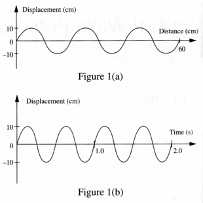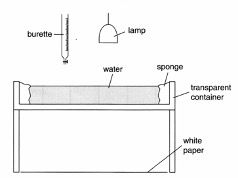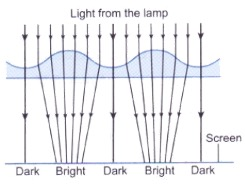# O Level Physics Core Topics – Waves

## 26 Jan O Level Physics Core Topics – WavesO Level Physics Core Topics – Waves

Main Components and Abstract Concepts

The main components of the Waves topic include describing properties of wave motion, key wave terminologies and the differences between types of waves. This is done through studying real life examples of wave motion, including vibrations in ropes and ripples along the water surface.

Students will be taught on the definitions of the aforementioned terminologies and how to identify these quantities in a given picture or figure. Students will be taught of the relationships between these quantities as well as relevant formulas, and how to represent these quantities and behaviour on suitable graphs.

Students are taught on how to apply concepts and formulas in solving related problems.

These concepts prepare the students for upcoming topics such as sound and electromagnetic waves as they are also similar applied there as well.

Electromagnetic (EM) waves are a group of waves which propagates through pertubations in the electromagnetic field. The topic discusses shared characteristics of all EM waves, arrangement of the EM spectrum and individual properties and applications of each component of the EM spectrum.

### What skills/techniques should the student have in order to master these two topics well?

Students need to have a precise understanding of the definition of the newly introduced terminologies so as to be able to apply it practically in given questions. Students need to be able to differentiate between the different types of graphs that can be presented to them, i.e. displacement-time graph displacement-distance graphs. With proper understanding of the concepts, students should be able to identify which variables are available to them from the individual graphs and hence able to read the graphs more accurately and effectively. After mastering this first step, the student can then move on to apply the wave equations to calculate for other unknowns if needed. This is essential as it is the fundamentals required for other chapters that revolves around waves.

Example 1Figure 1(a) is the displacement-distance graph and figure 1(b) is the displacement-time graph of a wave travelling along a slinky spring.

(a) What is the wavelength?

(b) Calculate

(i) the period,

(ii) the frequency

(iii) the velocity of the wave.

In the first question of the given example, the students need to identify which graph they are supposed to focus on in order to find the wavelength. According to the definition, a wavelength is the length of one full wave. Hence, by knowing that wavelength is a measure of length, they should be focusing on figure 1(a) instead of 1(b). The graph however provides 60cm for the length of three full waves. As you only need the length of one wave for a wavelength, this must be divided by 3 and the result should be 20cm for a wavelength.

Similarly in the next part, students should recall the definition a period. A period is the time taken to complete one full wave. Since it is a measure of time, the graph that the students should be focusing on is the graph in figure 1(b). The graph however provides the time of 1.0s for two full waves. Students should hence divide it by 2 to get a period of 0.5s.

The question then proceeds to test the students on the ability to apply the wave equations. The frequency variable exists in both wave equations that were discussed. But since the period is already known, then

frequency=1/period

period=0.5s

frequency=10.5

frequency=2.0Hz

The velocity (part (b)(iii)) is then calculated using the other wave equation.

velocity=frequency x wavelength

frequency=2.0Hz

wavelength=20cm

velocity=2 x 20=40.0cm/s

Example 2The figure below shows a set-up which is used by a teacher to demonstrate the properties of water waves.

(a) The lamp is used to project the water waves onto the white paper. It is observed that bright and dark lines are formed on the paper. Explain how the lines are produced.

(b) What is the purpose of the sponge placed at the side of the container?

(c) Water droplets fall onto the water surface from the burette. Sketch the wave pattern observed on the paper. Indicate the directions of propagation of the waves clearly.

(d) Suppose 5 water droplets fall from the burette onto the water surface per second.

(i) What is the frequency of the wave produced?

(ii) What is the wave speed if the distance between two crests is 4 cm?

(e) Explain what you would do to

(i) increase the distance between two crests.

(ii) increase the amplitude of the wave.

(a) In the first part of the first question, the students are required to incorporate their understanding of refraction from the light chapter together with the continuous motion of a wave. In this case, the light shines downwards from the lamp and will be incident at different angles at different point on the wave. This will then cause the rays of light to converge at specific points on the white paper, creating a series of light and dark spots. More specifically, the crests of the wave act as convex lenses which converges the light from the lamp to form bright lines. In two dimensions, the converging of light will look as such.(b) The students are also taught that waves reflect off a surface. The harder the surface, the more energy of the wave will be reflected off the surface. The softer the surface, the more energy of the wave will be absorbed by the surface instead. In the next part of the question, students are to apply that concept and identify that the purpose of the sponge is to absorb the energy of the incoming waves so as to prevent reflections from the edge which would disrupt the wave demonstration.

(c) In the next part, students are required to sketch the wave pattern that results from the fall of water droplets. Conceptually, the wave should propagate away from the source of disturbance. Above that, it should be identified that the setup is three dimensional. The resultant sketch should look something like this.

(d)(i) In this question, the first thing that the students are required to apply is their understanding of the definition of frequency. Five water droplets per second indicates that there are five waves per second which is saying that the frequency is 5Hz.

(ii) Distance between two crests is the definition of wavelength. The wavelength is therefore 4cm. To find the wave speed, the wave equation wave speed=frequency*wavelength can be used. The wave speed is therefore 20cm/s.

(e)(i) The distance between two crests is the definition of wavelength. According to the wave equation, the frequency and the wavelength are inversely proportional. Hence, to increase the wavelength, the frequency of the wave has to be decreased, i.e. decrease the number of water droplets per second.

(ii) The amplitude of the wave is related to the energy of the wave. A wave with a higher energy will have larger amplitude. This energy is the result of the energy that the water droplets carry. Therefore, the conversion of energy that takes place in this case is Gravitational potential energy🡪Kinetic Energy🡪Wave Energy. Hence, in order to increase the amplitude, the initial gravitational potential energy has to be increased, i.e. the water droplets must be dropped from a greater height.

How does Einstein help the students to conquer this topic?

At Einstein, we do not just drill on the concepts and definitions. Instead, students are taught on how these concepts relate to each other, their underlying relationships and explanations.  This ensures that they fully understand the concepts that are taught to them and are able to effectively apply them in the questions.

Students are guided through detailed thinking process in order to better comprehend the questions and tackle them more confidently. With the correct guidance and encouragement, the students will master the effective answering techniques with sound analytical skills. Contact Einstein now to hear from us more about the O level Physics program especially on how we can turbo charge your child’s mastery in Physics with renewed confidence and capabilities!